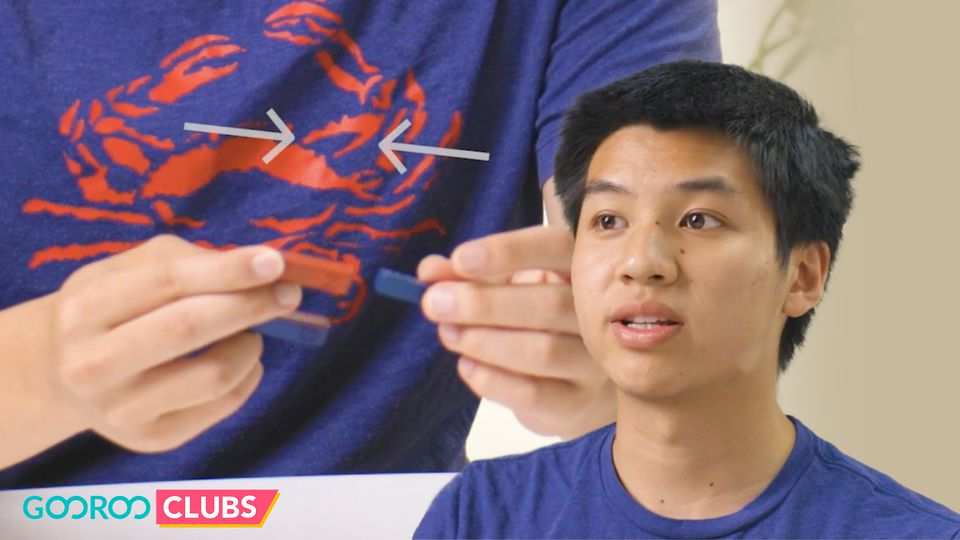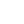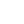Preview the Course
18m

# Forces and Interactions: Introduction to Force

by Max S. | ScienceLearning Standards: MS.PS2.1 MS.PS2.2 MS.PS2.3 MS.PS2.1; MS.PS2.2; MS.PS2.3;
7 Lessons 18:06
1.1. Weekly Learning Plan Trailer 0:36
2.2. The Forces Around Us 2:55
3.3. Rules of Force 2:55
4.4. Measuring Force 2:15
5.5. Interaction of forces 4:21
6.6. Measuring Gravity 1:53
7.7. How much would you weigh on a different planet? 3:11

18m

NGSS Standards

Have you ever wondered how we move in space? What drives the push and pull on objects, and why should we care about it? Dive into the basic physics concept and fundamental laws of force! Discover how gravity works and why it keeps you on the ground, building a foundation for greater physics concepts.

Magnets, electricity, force, and friction - master the crucial concepts of Forces and Interactions!

### Project

Applying and Measuring Force

### Course overview

1

Weekly Learning Plan Trailer

2

The Forces Around Us

Students will acquire a basic definition of what force is and be able to identify examples of forces at work.

3

Rules of Force

Students will learn how force is governed by and relates to Newton’s three laws.

4

Measuring Force

Students will learn about directionality and magnitude of force and its relation to acceleration.

5

Interaction of forces

Students will understand what happens when two forces interact and be able to find net force.

6

Measuring Gravity

Students will gain a numerical measurement of the force of gravity on Earth and its relation to acceleration.

7

How much would you weigh on a different planet?

Students will be able to relate gravity to mass and distance.

See more#### Quiz

Assess your learning with a quiz.

Tags:

• Gravity
• Isaac Newton
• Laws of Force
• Physics
• Science

• Blocks
• Spring scale
• Pencil
• Paper

## Instructor

Max is a physics enthusiast and rising senior at Rutgers University. Academically, he is most intrigued by the intuitive and approachable nature of forces and mechanics. Outside of physics, Max likes to stay active and follow sports.

See full profile